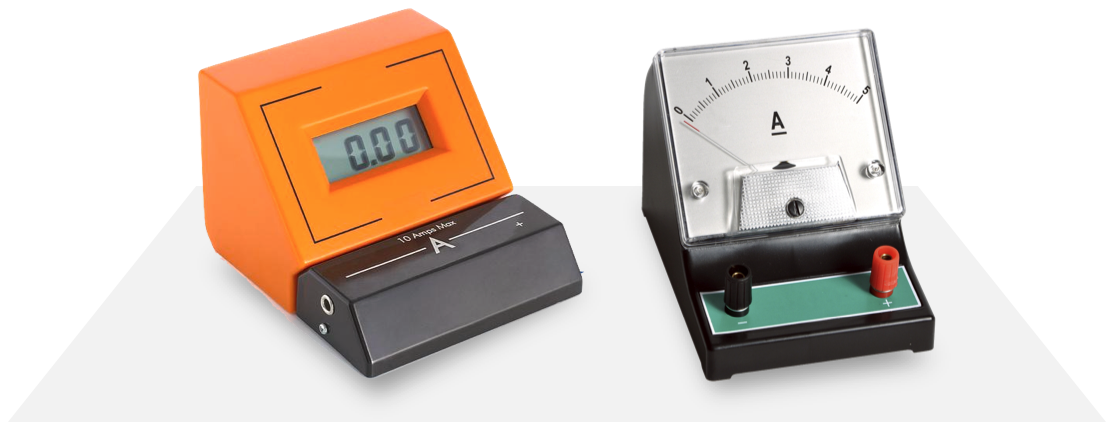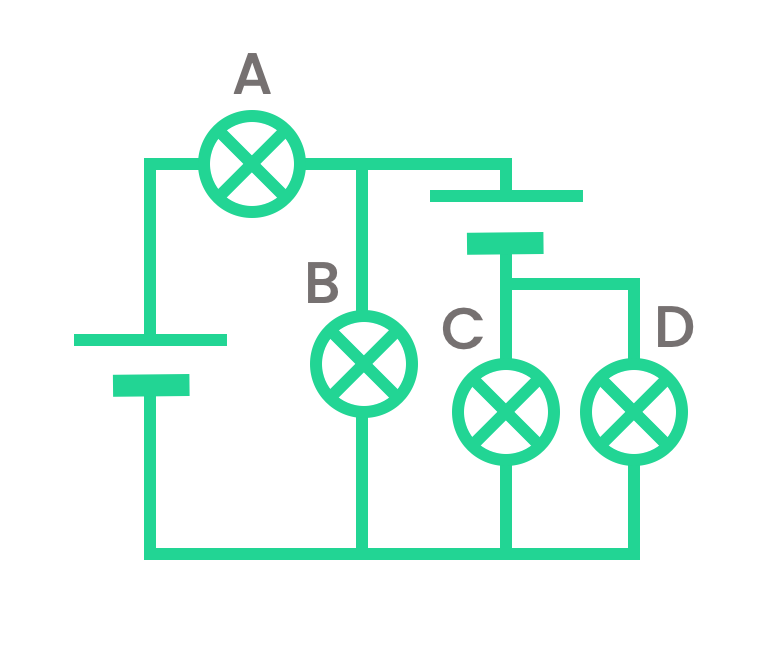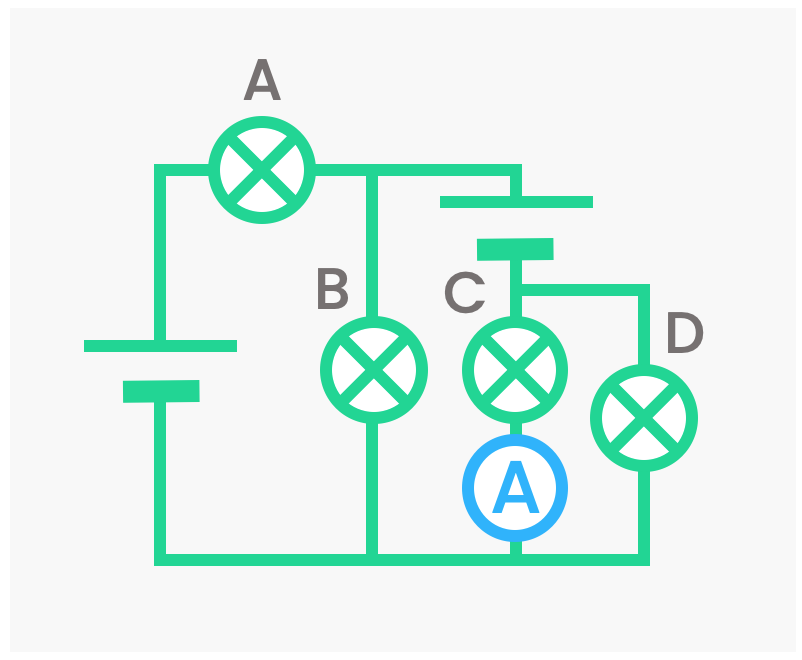Physics
Fox

### Summary

• An ammeter is used to measure the current flowing through any electrical component.• This is found by connecting an ammeter in series with the component.When messing around with circuits in the real world, we need to be able to measure the current and voltage of the circuit components (such as cells and bulbs). After all, what's physics if you can't measure things?

For measuring current, we use a device called an ammeter.

An ammeter measures the current through an electrical component. Here are a couple of examples you might find in a school lab:Left: A digital ammeter.
Right: An analogue ammeter.

This is the symbol for an ammeter, which we'll use on circuit diagrams:Here's a way to remember the name:
"measures Amps" → Amp-meter → Ammeter.

So how do we use an ammeter? It's easy, all we need to do is connect it in series with the electrical component we're interested in:Even in a complicated circuit like the one below, to measure the current through bulb C all we need to do is put an ammeter next to it.Instead of drawing an arrow to indicate current, we can instead draw an ammeter at that point. They mean exactly the same thing!An ammeter is connected in series with a bulb. The ammeter reads 5A, therefore the current through the bulb is 5A.

An ammeter doesn't actually measure the current through other components — it measures the current through itself!So how come it seems to measure the current through other components? Remember: All components which are in series always have the same current through them. So the current through an ammeter must be the same as any component it is in series with!

Quiz

### Congratulations!

7 of 7 questions completed

+ ⭐️ collected.

• You can change your display name at any time.
• at least 1 letter
• at least 1 number
• at least 8 characters